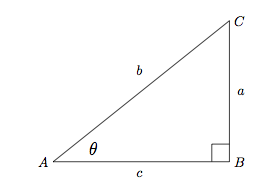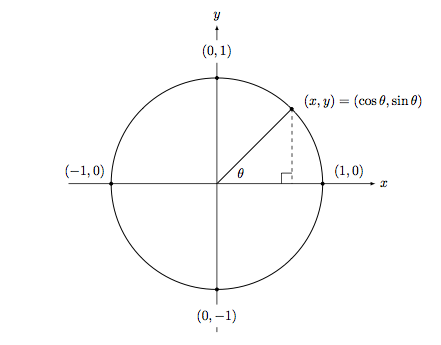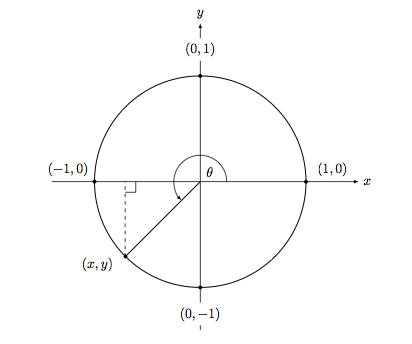# Trigonometric Functions

## Definition

The trigonometric functions relate the angles in a right triangle to the ratios of the sides. Given a triangle like this:Triangle ABC

The basic trigonometric functions would be defined, for $0^\circ < \theta < 90^{\circ}$ as:

$\begin{array}{lcr} \sin \theta = \frac{a}{b} & \cos \theta = \frac{c}{b} & \tan \theta = \frac{a}{c} \end{array}$

However, a more useful definition comes from the "unit circle." If we describe a circle with a radius of 1 unit, centered at the origin, then the angle $\theta$ inside the circle describes a right triangle when when we drop a perpendicular to the $x$-axis from the point of intersection with the circle.Unit Circle

Notice that the right triangle so described has a hypotenuse equal to the radius of the circle, an adjacent side length of $x$, and an opposite side length of $y$. This gives rise naturally to the following refined definitions:

$\begin{array}{lcr} \sin \theta = \frac{y}{r} & \cos \theta = \frac{x}{r} & \tan \theta = \frac{y}{x} \end{array}$

In the unit circle, as shown, since the radius is 1, this simplifies to $\sin \theta = y$, etc.

These definitions have the advantage of being compatible with the triangle definition above as well as allowing the evaluation of angles corresponding to any real number.

There are certain values of these functions which are useful to remember. They are:

$\begin{array} {| c | c | c | c | c | c |} \hline \theta & 0^\circ & 30^\circ & 45^\circ & 60^\circ & 90^\circ\\ \hline \sin \theta & \frac {\sqrt{0}} {2} & \frac {\sqrt{1}} {2} & \frac {\sqrt{2}} {2} & \frac {\sqrt{3}} {2} & \frac {\sqrt{4}} {2} \\ \hline \cos \theta & \frac {\sqrt{4}} {2} & \frac {\sqrt{3}} {2} & \frac {\sqrt{2}} {2} & \frac {\sqrt{1}} {2} & \frac {\sqrt{0}} {2}\\ \hline \tan \theta & 0 & \frac { 1}{\sqrt{3} } & 1 & \sqrt{3} & \mbox{undefined} \\ \hline \end{array}$

The reason for writing them in this way, is to aid remembering these terms. For example, the numerator for $\sin \theta$ is simply the square root of 0, 1, 2, 3, 4.

## Technique

### Evaluate $\sin 225^\circ$ exactly.Unit Circle with angle greater than 2 pi

Notice that the problem reduces to finding the value of $y$ in the above picture.

Since $225^\circ-180^\circ = 45^\circ$, $\theta$ makes an angle of $45^\circ$ with the negative $x$-axis. Further, since this is a right triangle, the angles must be $90^\circ + 45^\circ + 45^\circ = 180^\circ$, which means it is isosceles. Therefore, $| x | = |y|$.

By the Pythagorean Theorem, \begin{aligned} x^2 + y^2 &= 1 \\ 2y^2 &= 1 \\ y^2 &= \frac{1}{2} \\ y &= \pm \sqrt{\frac{1}{2}}=\pm \frac{\sqrt{2}}{2} \end{aligned}

By inspection, $y$ is negative, so $\sin 225^\circ = -\frac{\sqrt{2}}{2}$.

Note: most values for $\theta$ will be difficult or impossible to evaluate exactly in this way, so we often use a calculator to evaluate the approximate value of the function (see the table above for more exact values).

## Applications and Extensions

### Surveying

A surveyor in a helicopter at an elevation of 1000 meters measures the angle of depression to the far edge of an island as $24^\circ$ and the angle of depression to the near edge is $31^\circ$. How wide is the island, to the nearest meter?

Solution

Let the horizontal distance between the helicopter and the island be $d$, and the width of the island be $w$. Then $\tan 24^\circ = \frac{1000}{d+w}$ and $\tan 31^\circ = \frac{1000}{d}$. Thus $d = \frac{1000}{\tan 31^\circ}$. Substituting:

\begin{aligned} \tan 24^\circ &= \frac{1000}{\frac{1000}{\tan 31^\circ}+w} \\ \tan 24^\circ \left(\frac{1000}{\tan 31^\circ}+w \right) &= 1000 \\ w &= \frac{1000\left( 1 - \frac{\tan 24^\circ}{ \tan 31^\circ }\right)}{\tan 24^\circ} \\ w & \approx 581.75 \end{aligned}

Thus the width of the island is 582 meters.Note by Arron Kau
7 years, 1 month ago

This discussion board is a place to discuss our Daily Challenges and the math and science related to those challenges. Explanations are more than just a solution — they should explain the steps and thinking strategies that you used to obtain the solution. Comments should further the discussion of math and science.

When posting on Brilliant:

• Use the emojis to react to an explanation, whether you're congratulating a job well done , or just really confused .
• Ask specific questions about the challenge or the steps in somebody's explanation. Well-posed questions can add a lot to the discussion, but posting "I don't understand!" doesn't help anyone.
• Try to contribute something new to the discussion, whether it is an extension, generalization or other idea related to the challenge.

MarkdownAppears as
*italics* or _italics_ italics
**bold** or __bold__ bold
- bulleted- list
• bulleted
• list
1. numbered2. list
1. numbered
2. list
Note: you must add a full line of space before and after lists for them to show up correctly
paragraph 1paragraph 2

paragraph 1

paragraph 2

[example link](https://brilliant.org)example link
> This is a quote
This is a quote
    # I indented these lines
# 4 spaces, and now they show
# up as a code block.

print "hello world"
# I indented these lines
# 4 spaces, and now they show
# up as a code block.

print "hello world"
MathAppears as
Remember to wrap math in $$ ... $$ or $ ... $ to ensure proper formatting.
2 \times 3 $2 \times 3$
2^{34} $2^{34}$
a_{i-1} $a_{i-1}$
\frac{2}{3} $\frac{2}{3}$
\sqrt{2} $\sqrt{2}$
\sum_{i=1}^3 $\sum_{i=1}^3$
\sin \theta $\sin \theta$
\boxed{123} $\boxed{123}$

Sort by:

Tan 45-a/2

- 6 years, 8 months ago